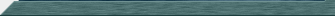n queens ``` queens.cpp - Solving the 'n' queens problem This program is a generalization of the classic computer science problem: How many ways can 8 queens be arranged on a chess board such that no queen can capture any of the others? Here is an example of a solution to the 8 queens problem [ ][ ][ ][ ][Q][ ][ ][ ] [ ][ ][ ][ ][ ][ ][Q][ ] [ ][Q][ ][ ][ ][ ][ ][ ] [ ][ ][ ][ ][ ][Q][ ][ ] [ ][ ][Q][ ][ ][ ][ ][ ] [Q][ ][ ][ ][ ][ ][ ][ ] [ ][ ][ ][Q][ ][ ][ ][ ] [ ][ ][ ][ ][ ][ ][ ][Q] Note that no row or column contains more than one queen and that there are no diagonal collisions either. The "no row or column contains more than one queen" gives a hint as to how to attack this problem. Consider a row of cells containing the numbers from 1 -> 8.  cell number: 1 2 3 4 5 6 7 8 Treating the cell number as the column and the contained value as the row, we can see that the above cells correspond the the 8 queens solution shown above. Note that the row of cells is simply a permutation of the numbers from 1 -> 8. Given that no two queens can share a row or a column, the set of all permutations of the numbers from 1 -> 8 represent a set of possible solutions to the 8 queens puzzle. Of course, we'll have to ensure that each permutation does not produce diagonal queen collisions but we've now reduced this problem from a truly staggering number of possible solutions: (64 * 63 * 62 * 61 * 60 * 59 * 58 * 57) --------------------------------------- => 4,426,165,368 (8 * 7 * 6 * 5 * 4 * 3 * 2) To a far more manageable number: 8! => (8 * 7 * 6 * 5 * 4 * 3 * 2) => 40,320 So, instead of investigating more than 4 billion possible solutions, we've reduced that number down by more than 100,000 times to a more manageable 40,320. For each candidate solution, to check the diagonals we start with an empty chess board: [ ][ ][ ][ ][ ][ ][ ][ ] [ ][ ][ ][ ][ ][ ][ ][ ] [ ][ ][ ][ ][ ][ ][ ][ ] [ ][ ][ ][ ][ ][ ][ ][ ] [ ][ ][ ][ ][ ][ ][ ][ ] [ ][ ][ ][ ][ ][ ][ ][ ] [ ][ ][ ][ ][ ][ ][ ][ ] [ ][ ][ ][ ][ ][ ][ ][ ] And as we examine the position of each queen we "mark off" all the diagonals representing spaces she could capture. Using our earlier solution, going position-by-position where 'x' represents a capturable position ... [ ][ ][ ][ ][ ][x][ ][ ] [ ][ ][ ][ ][x][ ][ ][ ] [ ][ ][ ][x][ ][ ][ ][ ] [ ][ ][x][ ][ ][ ][ ][ ] [ ][x][ ][ ][ ][ ][ ][ ] [Q][ ][ ][ ][ ][ ][ ][ ] [ ][x][ ][ ][ ][ ][ ][ ] [ ][ ][x][ ][ ][ ][ ][ ] [ ][ ][ ][x][ ][x][ ][ ] [x][ ][x][ ][x][ ][ ][ ] [ ][Q][ ][x][ ][ ][ ][ ] [x][ ][x][ ][ ][ ][ ][ ] [ ][x][ ][x][ ][ ][ ][ ] [Q][ ][ ][ ][x][ ][ ][ ] [ ][x][ ][ ][ ][x][ ][ ] [ ][ ][x][ ][ ][ ][x][ ] and so on through all 8 positions. Because no queen's position lands on an 'x', we've found a solution. To compile this program: unix: g++ -W -Wall -o queens queens.cpp win32: cl -W3 -GX queens.cpp Running the program without arguments presents solutions to the 8 queens problem, if run with a positive integer argument 'N' then solutions to the 'N' queens problem are presented. ```Source CodeReturn to Main